Win up to 100% scholarship on Aakash BYJU'S JEE/NEET courses with ABNAT Win up to 100% scholarship on Aakash BYJU'S JEE/NEET courses with ABNAT

# JEE Main 2022 June 28 – Shift 1 Chemistry Question Paper with Solutions

Students can refer to the JEE Main 2022 June 28 Shift 1 Chemistry question paper and solutions provided below. These solutions are written by our subject experts to help students understand the logic applied in getting the right answers. Also, you can get solutions for all JEE main 2022 question papers on our website. All the JEE question papers are solved in an easily understandable way. Students can start solving these question papers and verify the JEE main answer keys to evaluate their performance. Go through the solutions for the June 28 Shift 1 – JEE main 2022 chemistry question paper here.

## JEE Main 2022 June 28th Chemistry Shift 1 Question Paper and Solutions

#### SECTION – A

Multiple Choice Questions: This section contains 20 multiple choice questions. Each question has 4 choices (1), (2), (3) and (4), out of which ONLY ONE is correct.

1. The incorrect statement about the imperfections in solids is:

(A) Schottky defect decreases the density of the substance.

(B) Interstitial defect increases the density of the substance.

(C) Frenkel defect does not alter the density of the substance.

(D) Vacancy defect increases the density of the substance.

Sol. Vacancy defect causes decrease in density.

2. The Zeta potential is related to which property of colloids?

(A) Colour

(B) Tyndall effect

(C) Charge on the surface of colloidal particles

(D) Brownian movement

Sol. The potential difference between the fixed layer and the diffused layer of opposite charges is called zeta potential.

It is related with the charge on the surface of colloidal particles.

3. Element “E” belongs to the period 4 and group 16 of the periodic table. The valence shell electron configuration of the element, which is just above “E” in the group is

(A) 3s2, 3p4

(B) 3d10, 4s2, 4p4

(C) 4d10, 5s2, 5p4

(D) 2s2, 2p4

Sol. Element E is Selenium

The element which is just above ‘E’ in periodic table is sulphur, its electronic configuration is 1s2, 2s2, 2p6, 3s2, 3p4.

4. Given are two statements one is labelled as Assertion A and other is labelled as Reason R.

Assertion A : Magnesium can reduce Al2O3 at a temperature below 1350°C, while above 1350°C aluminium can reduce MgO.

Reason R : The melting and boiling points of magnesium are lower than those of aluminium.

In light of the above statements, choose the most appropriate answer from the options given below :

(A) Both A and R are correct, and R is correct explanation of A.

(B) Both A and R are correct, but R is NOT the correct explanation of A.

(C) A is correct R is not correct.

(D) A is not correct, R is correct.

Sol. Magnesium can reduce Al2O3 at a temperature below 1350°C while above 1350°C aluminium can reduce MgO because below 1350°C ΔG of MgO (formation) is more negative and above 1350°C ΔG of Al2O3 (formation) is more negative.

The melting and boiling point of magnesium are lower than those of aluminium but it is not the correct reason.

5. Dihydrogen reacts with CuO to give

(A) CuH2

(B) Cu

(C) Cu2O

(D) Cu(OH)2

Sol. CuO + H2 → Cu + H2O

6. Nitrogen gas is obtained by thermal decomposition of

(A) Ba(NO3)2

(B) Ba(N3)2

(C) NaNO2

(D) NaNO3

Sol.

$$\begin{array}{l}Ba\left ( N_3 \right )_2\xrightarrow[]{~~~\Delta~~~}Ba+3N_2\end{array}$$

7. Given below are two statements :

Statement I : The pentavalent oxide of group-15 element, E2O5, is less acidic than trivalent oxide, E2O3, of the same element.

Statement II : The acidic character of trivalent oxide of group 15 elements, E2O3, decreases down the group.

In light of the above statements, choose most appropriate answer from the options given below:

(A) Both Statement I and Statement II are true

(B) Both Statement I and Statement II are false

(C) Statement I true, but Statement II is false

(D) Statement I false, but Statement II is true

Sol. Statement I is false, as E2O5 is more acidic than E2O3

Statement II is correct.

8. Which one of the lanthanoids given below is the most stable in divalent form?

(A) Ce (Atomic Number 58)

(B) Sm (Atomic Number 62 )

(C) Eu (Atomic Number 63)

(D) Yb (Atomic Number 70)

Sol. Eu+2 is 4f7

Yb+2 is 4f14

but Eu+2 is more stable than Yb+2 because

$$\begin{array}{l}E_{Eu|Eu^{2+}}^\circ > E_{Yb|Yb^{2+}}^\circ\end{array}$$

9. Given below are two statements:

Statement I: [Ni(CN)4]2– is square planar and diamagnetic complex, with dsp2 hybridization for Ni but [Ni(CO)4] is tetrahedral, paramagnetic and with sp3-hybridization for Ni.

Statement II: [NiCl4]2– and [Ni(CO)4] both have same d-electron configuration, have same geometry and are paramagnetic.

In light of the above statements, choose the correct answer from the options given below :

(A) Both Statement I and Statement II are true

(B) Both Statement I and Statement II are false

(C) Statement I is correct but Statement II is false

(D) Statement I is incorrect but Statement II is true

Sol. [Ni(CN)4]2– is square planar and diamagnetic with μ = 0

its hybridisation is dsp2

Ni(CO)4 is tetrahedral but diamagnetic.

10. Which amongst the following is not a pesticide?

(A) DDT

(B) Organophosphates

(C) Dieldrin

(D) Sodium arsenite

Sol. Sodium arsenite is a herbicide.

11. Which one of the following techniques is not used to spot components of a mixture separated on thin layer chromatographic plate?

(A) I2 (Solid)

(B) U.V. Light

(C) Visualisation agent as a component of mobile phase

(D) Spraying of an appropriate reagent

Sol. The function of mobile phase is to carry the components present on TLC.

12. Which of the following structures are aromatic in nature?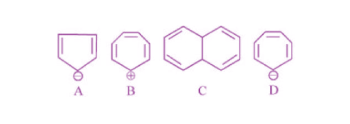(A) A, B, C and D

(B) Only A and B

(C) Only A and C

(D) Only B, C and D

Sol.are aromatic as they are cyclic , planar and has 4n + 2 π e (n = 1)

13. The major product (P) in the reaction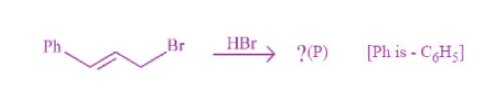is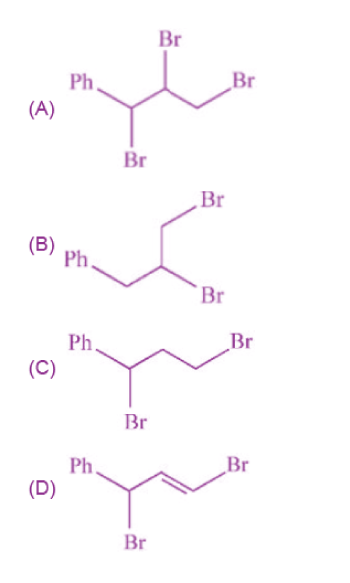Sol.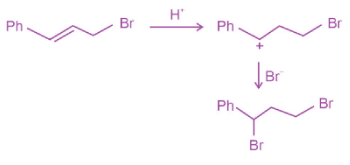14. The correct structure of product ‘A’ formed in the following reaction,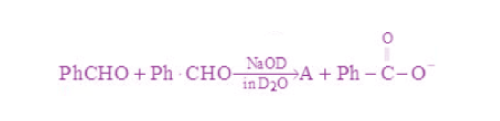(Ph is – C6H5) is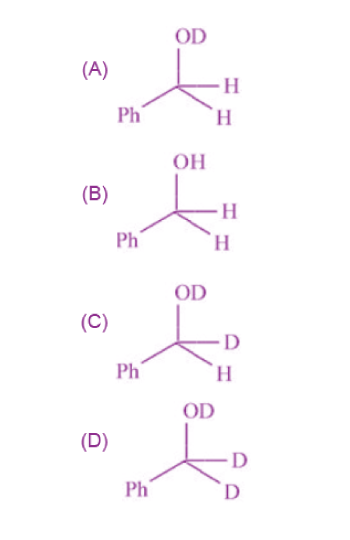Sol.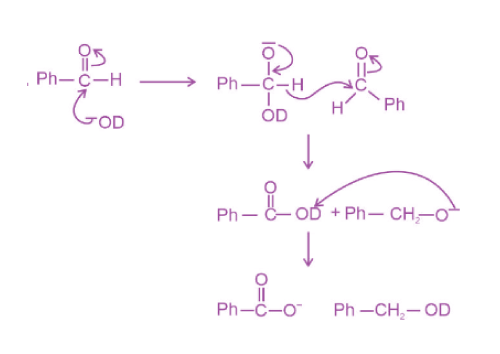15. Which one of the following compounds is inactive towards SN1 reaction?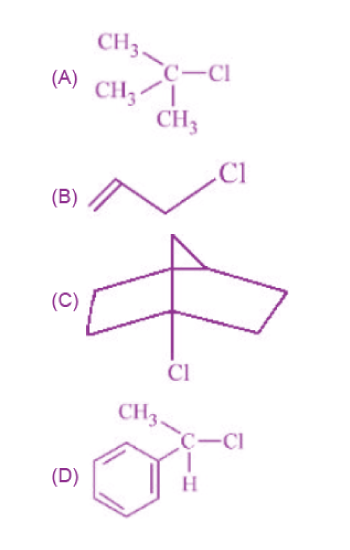Sol.is inactive towards SN1 as halogen is attached to bridge head carbon atom, where formation of carbocation is not possible.

16. Identify the major product formed in the following sequence of reactions: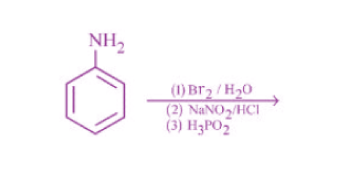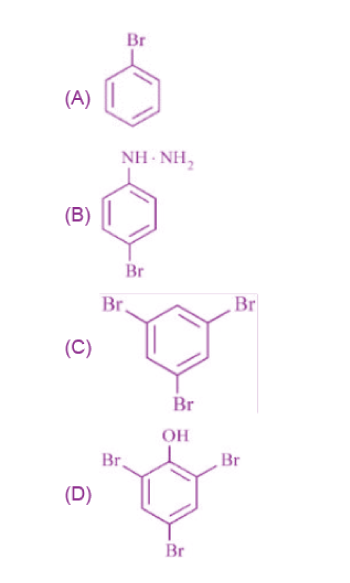Sol.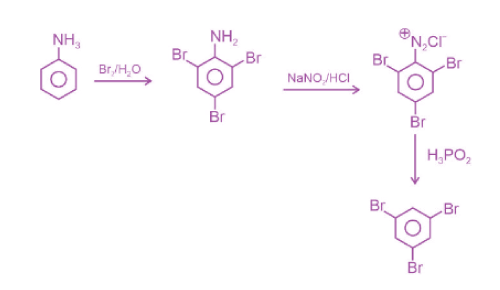17. A primary aliphatic amine on reaction with nitrous acid in cold (273 K) and there after raising temperature of reaction mixture to room temperature (298 K), gives

(A) nitrile

(B) alcohol

(C) diazonium salt

(D) secondary amine

Sol.18. Which one of the following is NOT a copolymer?

(A) Buna-S

(B) Neoprene

(C) PHBV

Sol. Monomer of neoprene is 2-chloro-1,3-butadiene, and it is not a copolymer.

19. Stability of α-Helix structure of proteins depends upon

(A) dipolar interaction

(B) H-bonding interaction

(C) van der Walls forces

(D) π-stacking interaction

Sol. Mostly H-bonding is responsible for the stability of α-helix form.

20. The formula of the purple colour formed in Laissaigne’s test for sulphur using sodium nitroprusside is

(A) NaFe[Fe(CN)6]

(B) Na[Cr(NH3)2(NCS)4]

(C) Na2[Fe(CN)5(NO)]

(D) Na4[Fe(CN)5(NOS)]

Sol. S2– + [Fe(CN)5NO)]2– → [Fe(CN)5(NOS)]4–

Purple

#### SECTION – B

Numerical Value Type Questions: This section contains 10 questions. In Section B, attempt any five questions out of 10. The answer to each question is a NUMERICAL VALUE. For each question, enter the correct numerical value (in decimal notation, truncated/rounded-off to the second decimal place; e.g. 06.25, 07.00, –00.33, –00.30, 30.27, –27.30) using the mouse and the on-screen virtual numeric keypad in the place designated to enter the answer.

1. A 2.0 g sample containing MnO2 is treated with HCl liberating Cl2. The Cl2 gas is passed into a solution of KI and 60.0 mL of 0.1 M Na2S2O3 is required to titrate the liberated iodine. The percentage of MnO2 in the sample is ______. (Nearest integer)

[Atomic masses (in u) Mn = 55; Cl = 35.5; O = 16, I = 127, Na = 23, K = 39, S = 32]

Sol. MnO2 + 4HCl → MnCl2 + 2H2O + Cl2

$$\begin{array}{l}Cl_2+Kl\to CL^{-}+I_2\xrightarrow{2Na_2S_2O_3}Na_2S_4O_6+2Nal\end{array}$$

Equivalent of MnO2 = HCl = Cl2 = I2 = Na2S2O3

2 × number of moles of MnO2 = 1 × number of moles of Na2S2O3

Moles of MnO2

$$\begin{array}{l}=\frac{60\times0.1\times10^{-3}}{2}\end{array}$$

= 3 × 10–3 mole

Mass of MnO2 = 0.261 g

% of MnO2

$$\begin{array}{l}=\frac{0.261}{2}\times100\simeq 13\%\end{array}$$

2. lf the work function of a metal is 6.63 × 10–19 J, the maximum wavelength of the photon required to remove a photoelectron from the metal is ______ nm. (Nearest integer)

[Given : h = 6.63 × 10–34 J s, and c = 3 × 108 m s–1]

Sol.

$$\begin{array}{l}\because E=\frac{hc}{\lambda}\end{array}$$
$$\begin{array}{l}6.63\times10^{-19}=\frac{6.63\times10^{-34}\times3\times10^8}{\lambda}\end{array}$$
$$\begin{array}{l}\lambda=3\times10^{-7}m \end{array}$$

= 300 × 10–9 m

= 300 nm

3. The hybridization of P exhibited in PF5 is spxdy. The value of y is _______

Sol. PF5 is sp3d hybridised

y = 1

4. 4.0 L of an ideal gas is allowed to expand isothermally into vacuum until the total volume is 20 L. The amount of heat absorbed in this expansion is ____ L atm.

Sol. Work done = –Pext Δv

∵ Pext = 0 (vacuum)

∴ w = 0, ΔU = 0 (as the process is isothermal)

So, q = 0

5. The vapour pressures of two volatile liquids A and B at 25°C are 50 Torr and 100 Torr, respectively. If the liquid mixture, contains 0.3 mole fraction of A, then the mole fraction of liquid B in the vapour phase is x/17. The value of x is _______.

Sol.

$$\begin{array}{l}P_T=P_A^0\cdot x_A+P_B^0\cdot x_B \end{array}$$

= 50 × 0.3 + 100 × 0.7

= 85 mm Hg

$$\begin{array}{l}y_B=\frac{70}{85}=\frac{x}{17}\end{array}$$
$$\begin{array}{l}\frac{x}{17}=14 \end{array}$$

6. The solubility product of a sparingly soluble salt A2X3 is 1.1 × 10–23. If the specific conductance of the solution is 3 × 10–5 S m–1, the limiting molar conductivity of the solution is x × 10–3 S m2 mol–1. The value of x is _______.

Sol.

$$\begin{array}{l}\begin{matrix}A_2X_3 & \rightleftharpoons & 2A & + & 3x \\ & & 2S & & 3S \\\end{matrix}\end{array}$$

Ksp = (2s)2(3s)3 = 1.1 × 10–23

$$\begin{array}{l}S\approx 10^{-5} \end{array}$$

For sparingly soluble salts

$$\begin{array}{l}\wedge_m=\wedge_m^\circ\end{array}$$
$$\begin{array}{l}\wedge_m=\frac{k}{S\times10^3}\end{array}$$
$$\begin{array}{l}=\frac{3\times10^{-5}}{10^{-5}}\times10^{-3} \end{array}$$
$$\begin{array}{l}=3\times10^{-3}~~\textup{Sm}^2~~\textup{mol}^{-1}\end{array}$$

7. The quantity of electricity of Faraday needed to reduce 1 mol of

$$\begin{array}{l}Cr_2O_{7-}^2 \end{array}$$
to Cr3+ is

Sol.

$$\begin{array}{l}\overset{+6}{C}r_2O_{7}^{2-} \xrightarrow[]{~~~~~} 2Cr^{3+}\end{array}$$

∵ Each Cr is converting from +6 to +3

∴ 6 faradays of charge is required

8. For a first order reaction A → B, the rate constant, k = 5.5 × 10–14 s–1. The time required for 67% completion of reaction is x × 10–1 times the half life of reaction. The value of x is _____ (Nearest integer)

Sol.

$$\begin{array}{l}\because kt = \text{In}\frac{A_0}{A}\end{array}$$
$$\begin{array}{l}\frac{In2}{t_\frac{1}{2}}t_{67\%}=In\frac{A_0}{0.33A_0}\end{array}$$
$$\begin{array}{l}\frac{log2}{t_\frac{1}{2}} t_{67\%}=log\frac{1}{0.33}\end{array}$$

t67% = 1.566 t1/2

x = 15.66

Nearest integer = 16

9. Number of complexes which will exhibit synergic bonding amongst, [Cr(CO)6], [Mn(CO)5] and [Mn2(CO)10] is ________.

Sol. CO ligand shows synergic bonding, so all complexes can show synergic bonding.

10. In the estimation of bromine, 0.5 g of an organic compound gave 0.40 g of silver bromide. The percentage of bromine in the given compound is _____% (nearest integer)

(Relative atomic masses of Ag and Br are 108 u and 80 u, respectively).

Sol. 188 g AgBr has 80 g of Br

∴ 0.4 g AgBr

$$\begin{array}{l}=\frac{80}{188}\times0.4 \end{array}$$

% of Br in given organic compound

$$\begin{array}{l}=\frac{80\times0.4}{188\times0.5}\times100 \end{array}$$

≈ 34%

### Download PDF of JEE Main 2022 June 28 Shift 1 Chemistry Paper & Solutions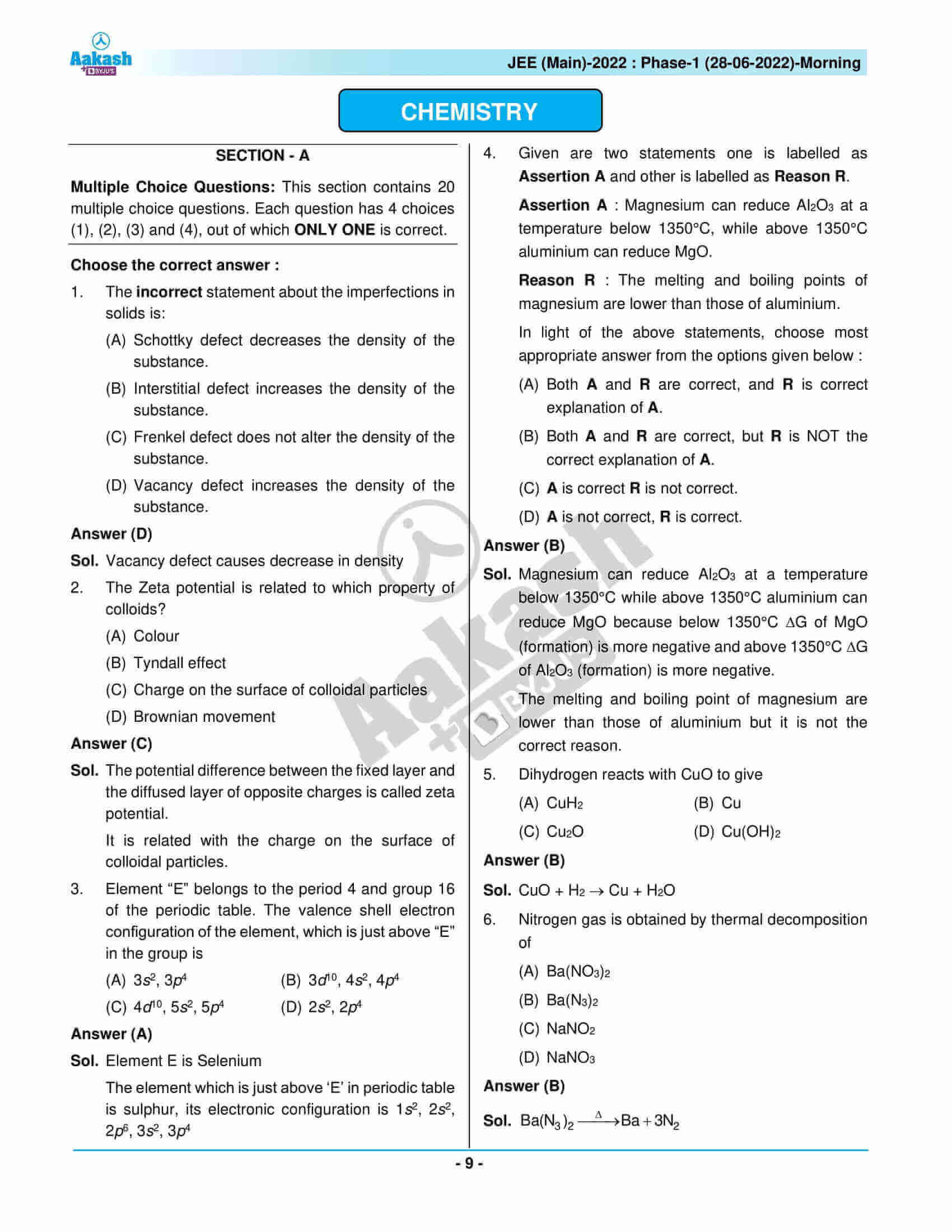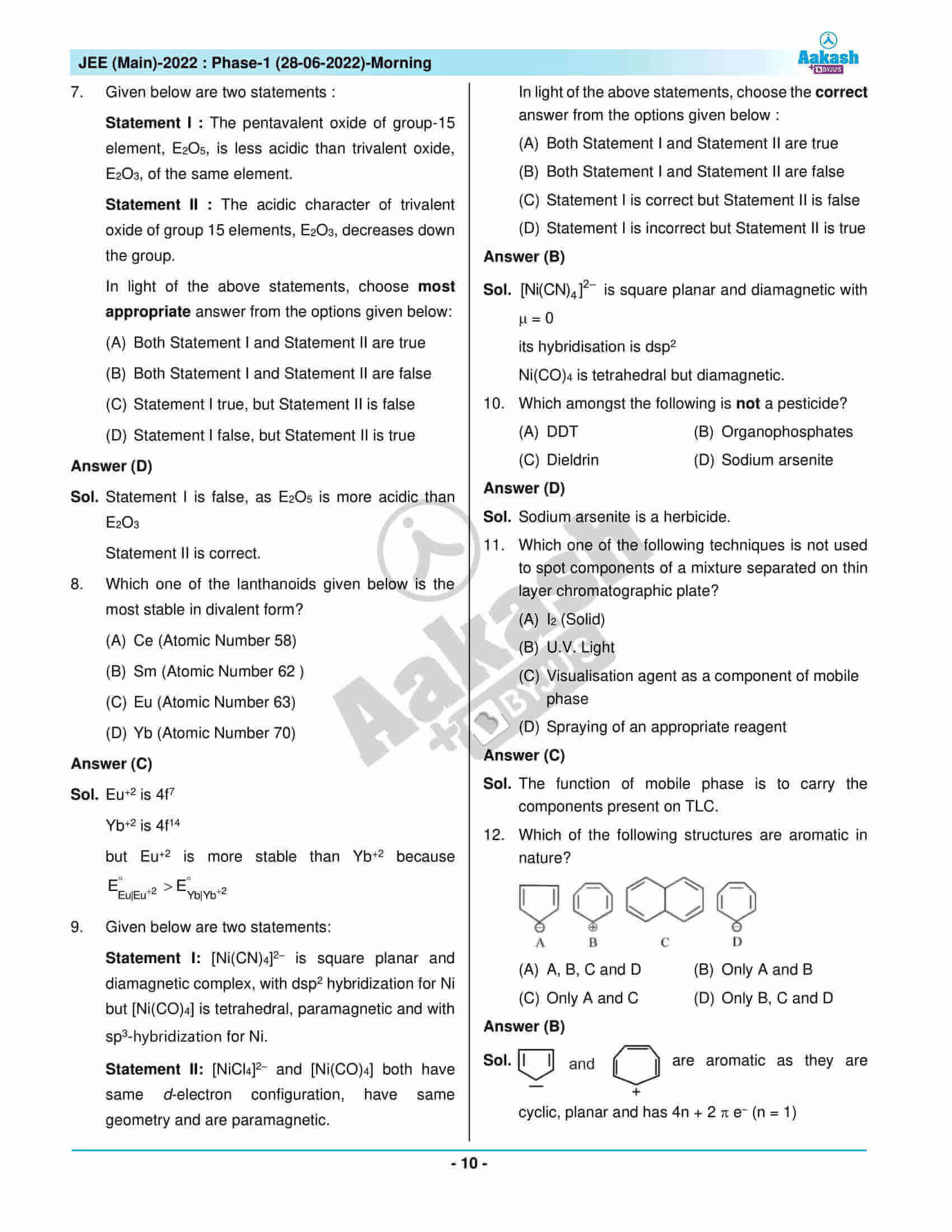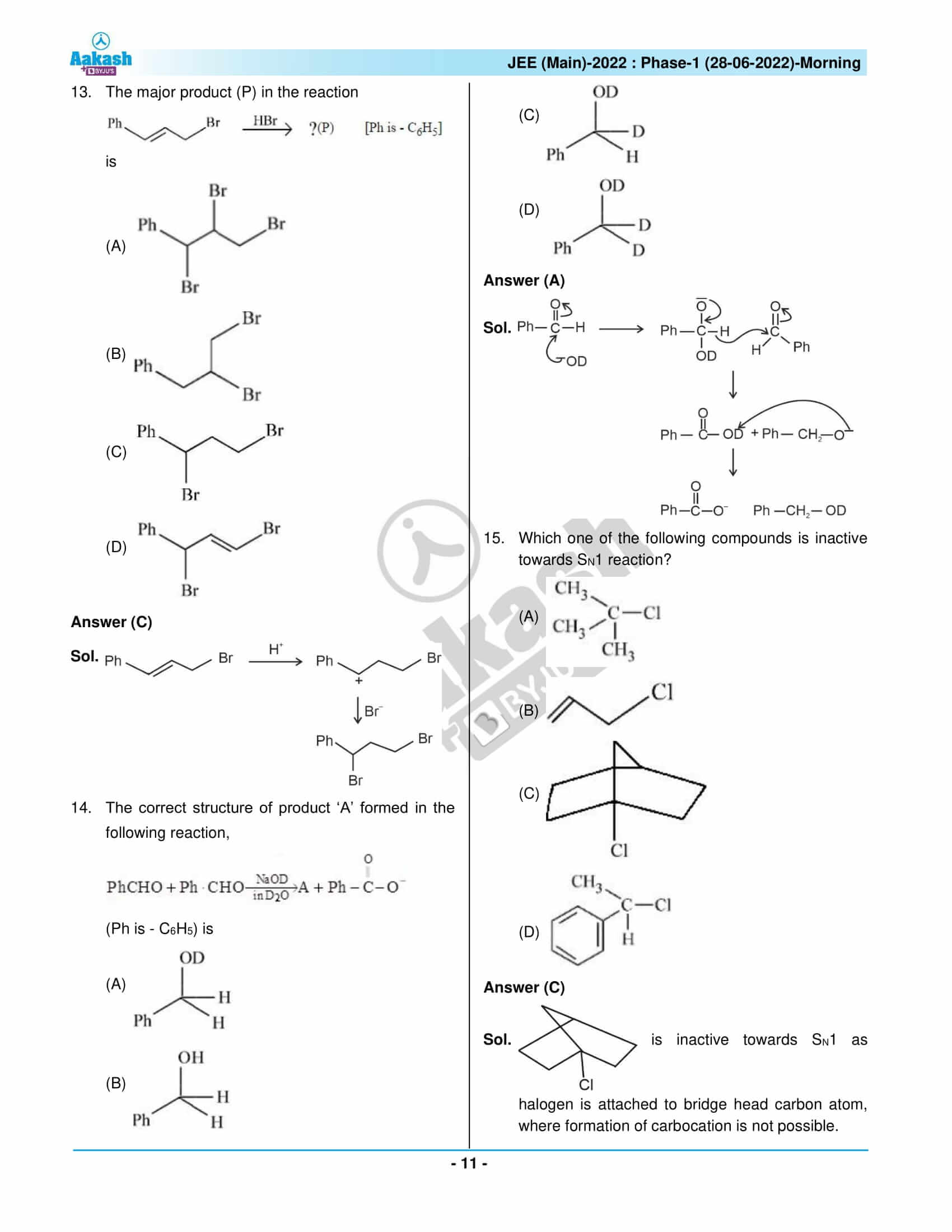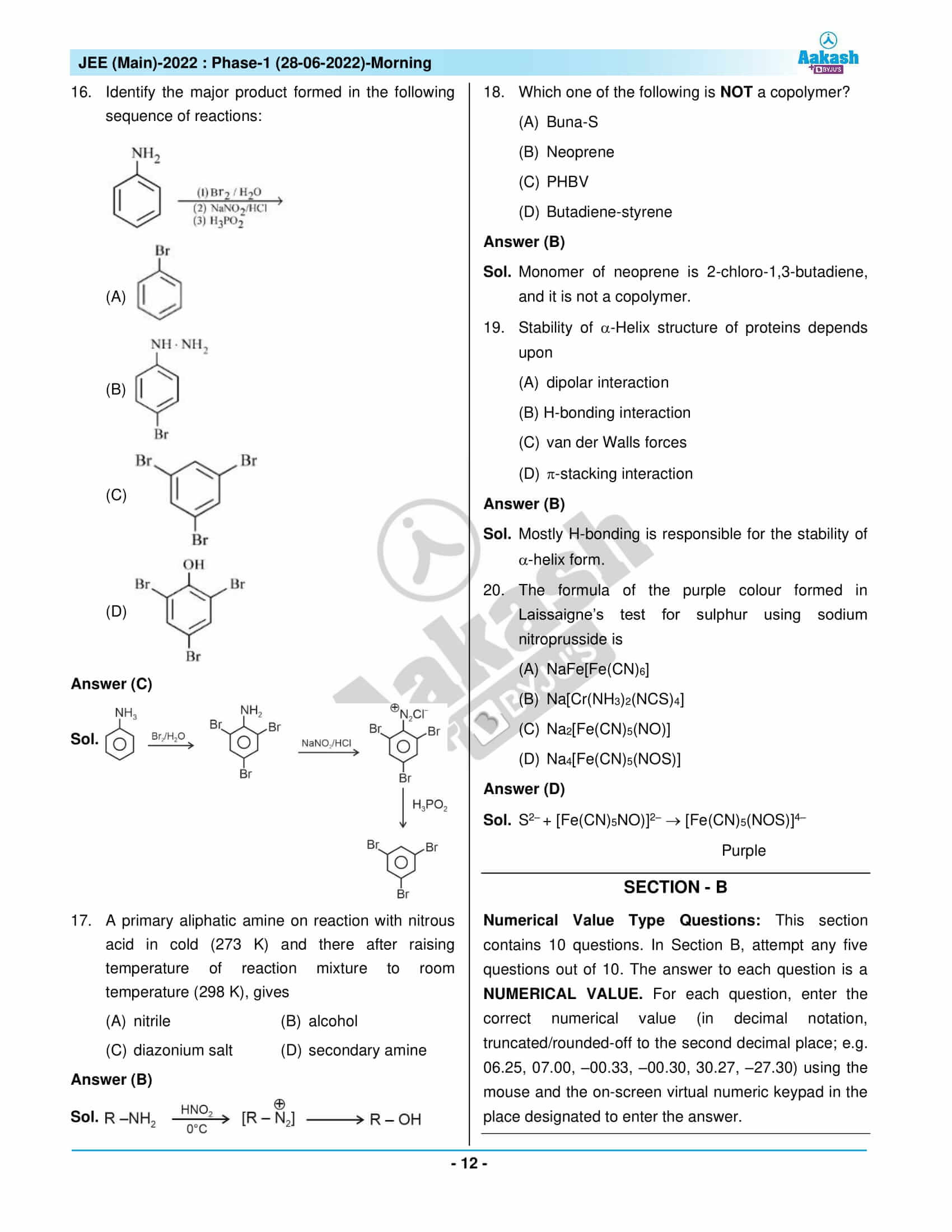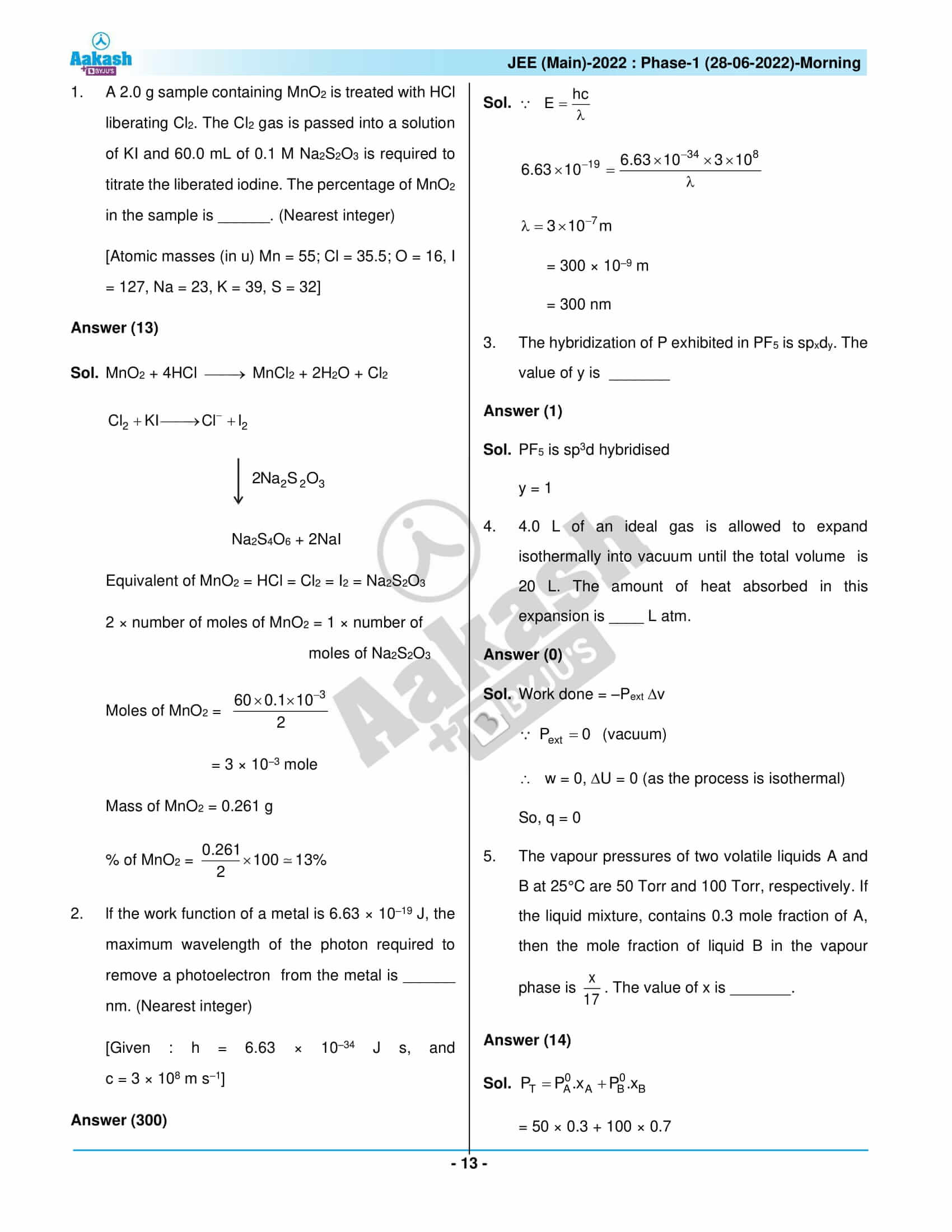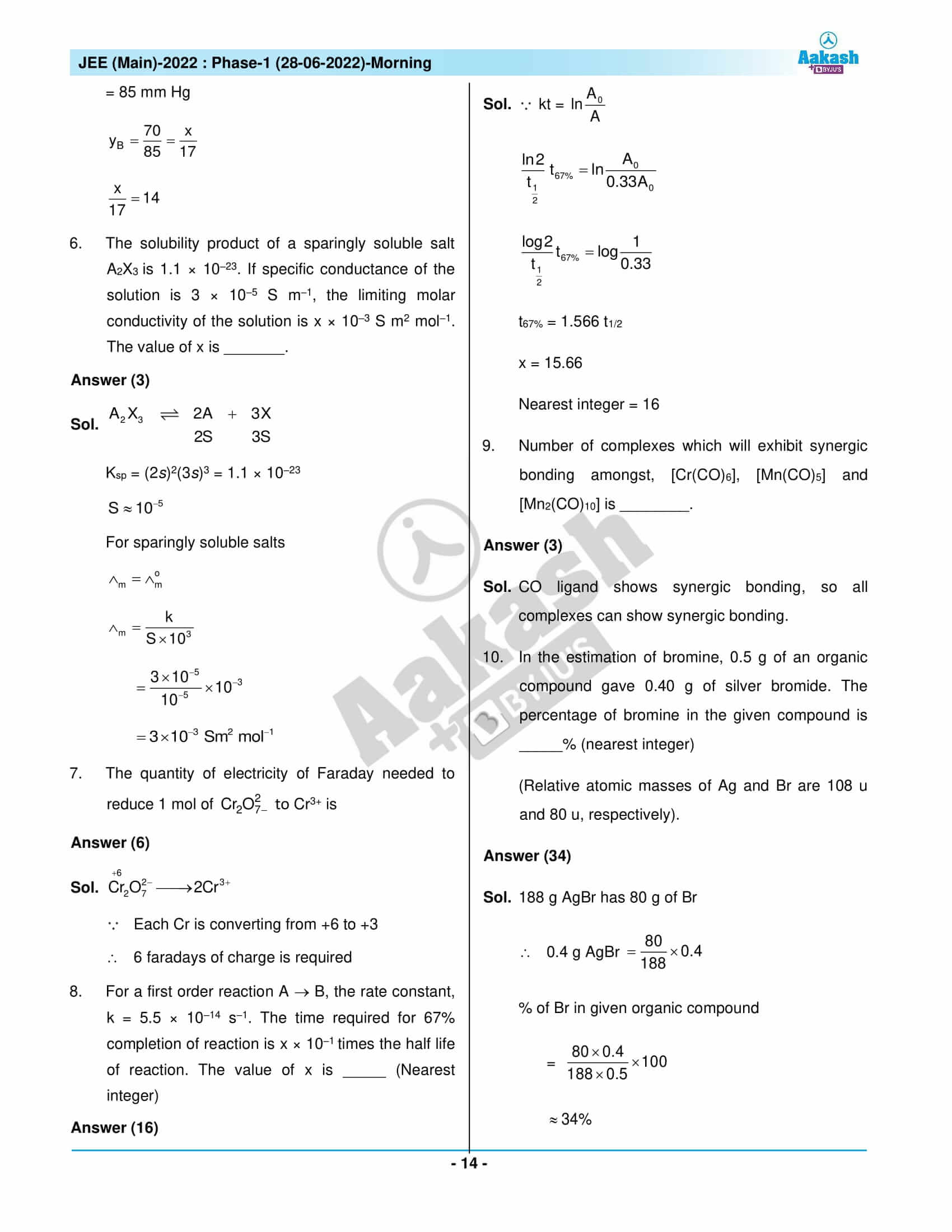## JEE Main 2022 June 28 Shift 1 Paper with Solutions

### JEE Main 2022 June 28 Shift 1 Question Paper – Physics Solutions### JEE Main 2022 June 28 Shift 1 Question Paper – Chemistry Solutions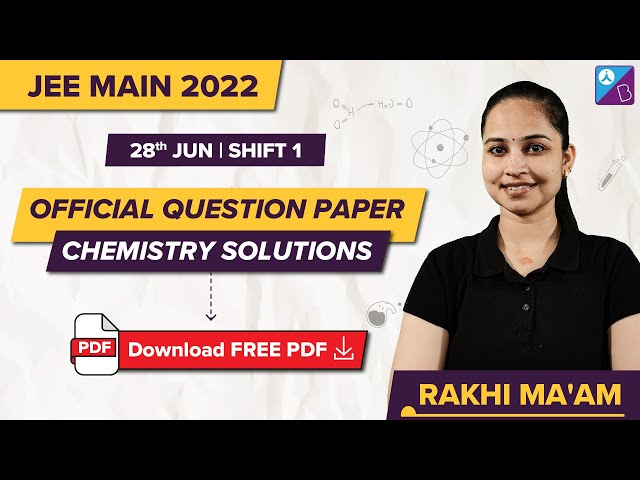### JEE Main 2022 June 28 Shift 1 Question Paper – Maths Solutions## Frequently Asked Questions – FAQs

Q1

### What is the difficulty level of the JEE Main 2022 28th June Shift 1 Chemistry question paper?

The JEE Main 2022 28th June Shift 1 Chemistry question paper contained an easy to moderate difficulty level of questions. There were 10 medium and 15 easy questions as per the memory-based question paper.

Q2

### Were there any questions from the chapter Solid state in JEE Main 2022 June 28 Shift 1 Chemistry question paper?

Yes. There were questions about defects in solids from the chapter Solid state in JEE Main 2022 June 28 Shift 1 Chemistry question paper.

Q3

### What is the total marks of the JEE Main 2022 June 28 Shift 1 Chemistry question paper?

The total marks of the JEE Main 2022 June 28 Shift 1 Chemistry question paper is 120.

Q4

### What is the difficulty level of questions asked from Biomolecules in the JEE Main 2022 Chemistry June 28 Shift 1 question paper?

The questions asked from Biomolecules in the JEE Main 2022 Chemistry June 28 Shift 1 question paper were easy.

Q5

### How many questions were there from Class 12 in the JEE Main 2022 Chemistry Shift 1 June 28 question paper?

There were 9 questions from Class 12 in the JEE Main 2022 Chemistry Shift 1 June 28 question paper as per the memory-based question paper.